## Quiz 3 : Linear ProgrammingLooking for Decision Science Homework Help?# Quiz 3 : Linear Programming

Consider the pizza slices as x, hot dogs as y, and barbeque sandwiches as z. Determine the profit obtained from selling each of the mentioned items as shown below: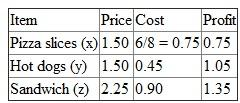a. Formulate the linear programming problem as shown below: Objective function is to maximize the profit of the food items. Maximize Z = 0.75x + 1.05y + 1.35z Subject to the following constraints: The space available is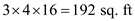which is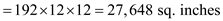The oven will be refilled during half time. Thus, the total space will be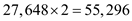Space required for pizza to keep warm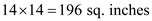Space required for pizza slice to keep warm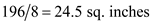Thus, frame this constraint as 24.5x +16y + 25z = 55,296. The total available purchasing cost is \$1,500. Cost per pizza slice is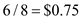Thus, 0.75x + 0.45y + 0.90z = 1,500 The seller can sell at least as many slices of pizza as hot dogs and barbecue sandwiches combined. Thus, x = y + z She can sell at least twice as many hot dogs as barbecue sandwiches. Thus, y =2z The linear programming model is given below: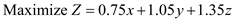Subject toEnter the values in the excel sheet as shown below: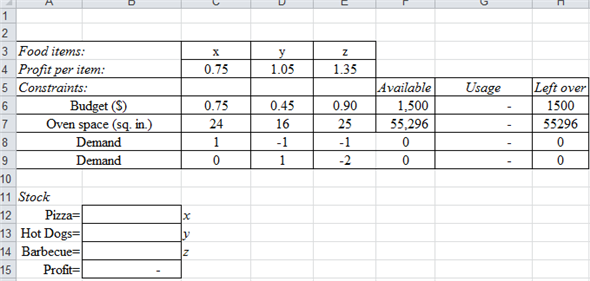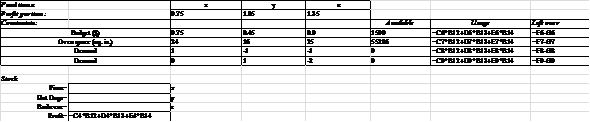Access the solver from the 'data-solver' menu as shown below: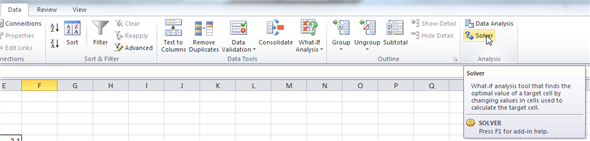Enter the values and constraints in the solver as shown below: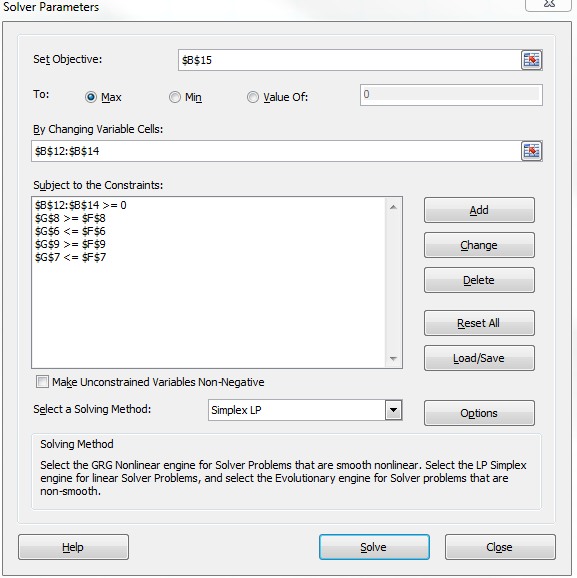The total profit she can earn is \$2,250 as shown below: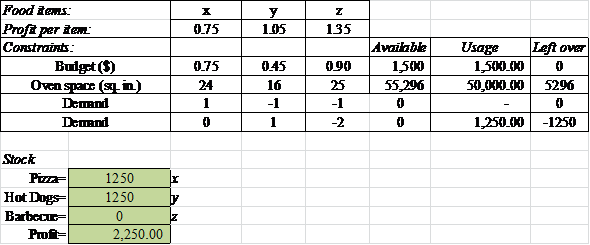Since, she is earning \$2,250 which is higher than the \$1,100. Thus, she can lease the booth. b) The sensitivity analysis for the linear program is given below: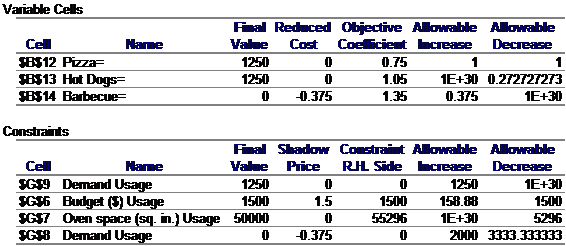The shadow price for the budget is \$1.5. This means each dollar added to the budget would increase the profit by \$1.5. The maximum allowable increase is \$158.88. Thus, she can borrow funds of \$158.88 which would make an additional profit by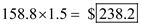. c) The lease cost for the booth per game is \$1,000 and the least cost for the oven per game is \$100. Thus, the total expense is \$1,100. The total profit is \$2,250. Calculate the net profit as shown below: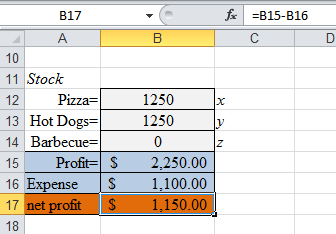Thus, the net profit is \$1,150.00. If J hires a friend to help her for \$100 per game, her net profit will reduce by \$100 which would give her \$1,050 net profit. She can hire her friend to assist her as per the strategy it is worth leasing the booth. d) The uncertain factor is the demand. If the demand for her products decreases she would lose the profit. She has a plan to hire her friend to assist her in producing hot dogs. She can withdraw this plan. This way even if her demand drops down she can still earn certain profit.

a) MTL is planning to produce two different types of tiles; large tiles and small tiles. Consider large tiles as x and smaller tiles as y. These are produced in batches of 100. Large tiles earn a profit of \$190 and a smaller tile earns a profit of \$240. Formulate the linear programming as shown below: \$190x + \$240y Subject to the following constants: 18x + 15y = 3600 0.27x + 0.58y = 105 0.16x + 0.20y = 40 32.8x + 20y = 6000 To determine the mix of tiles it should manufacture each week, use excel solver as shown below: Enter the values in the excel sheet as shown below: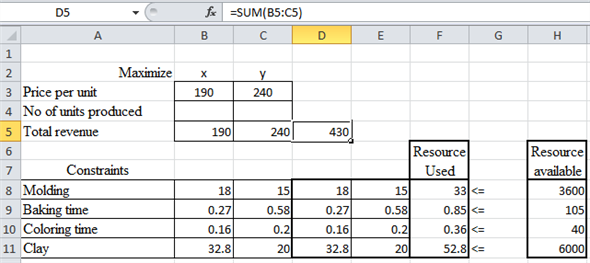Access 'Solver' from the 'data' menu in the excel sheet as shown below: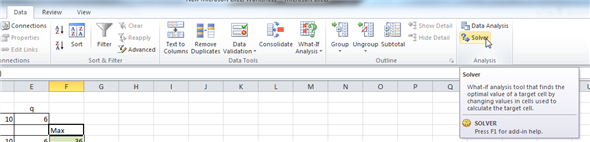Enter the objectives and the constraints in the solver as shown below: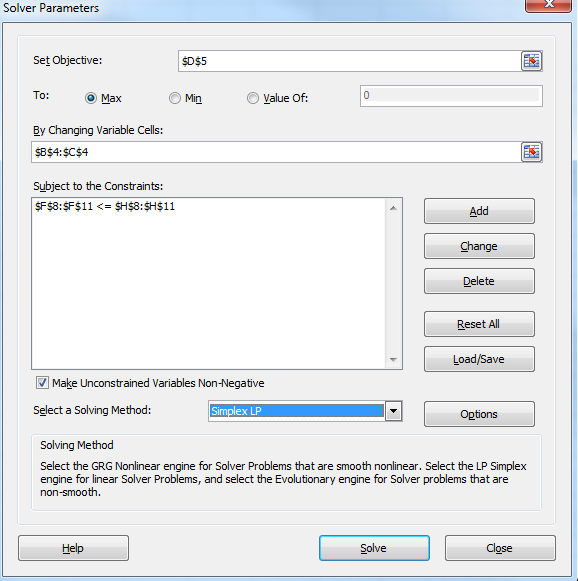The solver produces the following result: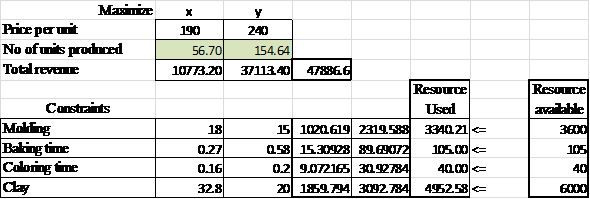Thus, 57 batches of larger tiles and 155 batches of smaller tiles must be produced to maximize the profit. b) The model in the standard form is as shown below: Max 190 x + 240 y Subject to the following constraints: Convert the inequalities into equalities using slack variables. 18x + 15y + S1 = 3600 0.27x + 0.58y + S2 = 105 0.16x + 0.20y +S3 = 40 32.8x + 20y + S4 = 6,000 c) Formulate the linear programming as shown below: \$190x + \$240y Subject to the following constants: 18x + 15y = 3600 …… (1) 0.27x + 0.58y = 105 …… (2) 0.16x + 0.20y = 40 …… (3) 32.8x + 20y = 6000 …… (4) Identify the values of X and Y coordinates for the equation.. …. (1) as shown below: 18x + 15y = 3600 Consider 'x' as 0 and calculate 'y' as shown below:Thus, y=240 in the equation …… (1). Consider 'y' as 0 and calculate 'x' as shown below:Thus, x=200 in the equation …… (1). The coordinates are (0, 240) and (200, 0). Identify the values of X and Y coordinates for the equation.. …. (2) as shown below: 0.27x + 0.58y = 105 Consider 'x' as 0 and calculate 'y' as shown below:Thus, y=181 in the equation …… (2). Consider 'y' as 0 and calculate 'x' as shown below:Thus, x=388.8 in the equation …… (2). The coordinates are (0, 181) and (388.8, 0). Identify the values of X and Y coordinates for the equation.. …. (3) as shown below: 0.16x + 0.20y = 40 Consider 'x' as 0 and calculate 'y' as shown below:Thus, y=200 in the equation …… (3). Consider 'y' as 0 and calculate 'x' as shown below:Thus, x=400 in the equation …… (3). The coordinates are (0, 200) and (400, 0). Identify the values of X and Y coordinates for the equation.. …. (4) as shown below: 32.8x + 20y = 6000 Consider 'x' as 0 and calculate 'y' as shown below:Thus, y=300 in the equation …… (4). Consider 'y' as 0 and calculate 'x' as shown below:Thus, x=182.92 in the equation …… (4). The coordinates are (0, 300) and (182.92, 0). The coordinates of 18x + 15y = 3600 are (0, 240) and (200, 0). The coordinates of 0.27x + 0.58y = 105 are (0, 181) and (388.8, 0). The coordinates of 0.16x + 0.20y = 40 are (0, 200) and (400, 0). The coordinates of 32.8x + 20y = 6,000 are (0, 300) and (182.92, 0). Draw the graph using the coordinates as shown below: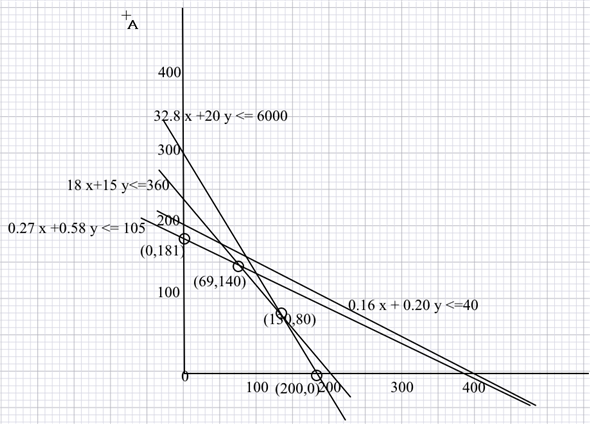The corner points are (0, 181), (69, 140), (130, 80), and (200, 0). Substitute the x and y coordinates in the maximize equation to find the optimal solution as shown below: Substitute x as 0 and y as 181Substitute x as 69 and y as 140Substitute x as 130 and y as 180Substitute x as 200 and y as 0The maximum value is the optimal solution which is 67,900. d) Molding time and the clay are not utilized completely as shown below: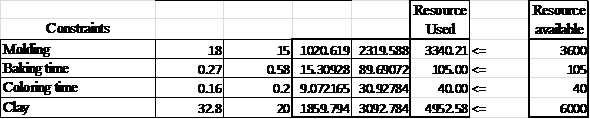e) The sensitivity ranges for the objective function and the constraints are as shown below: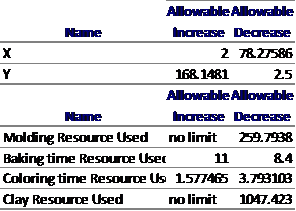F) Enter the values in the excel sheet as shown below:Access 'Solver' from the 'data' menu in the excel sheet as shown below:Enter the objectives and the constraints in the solver as shown below: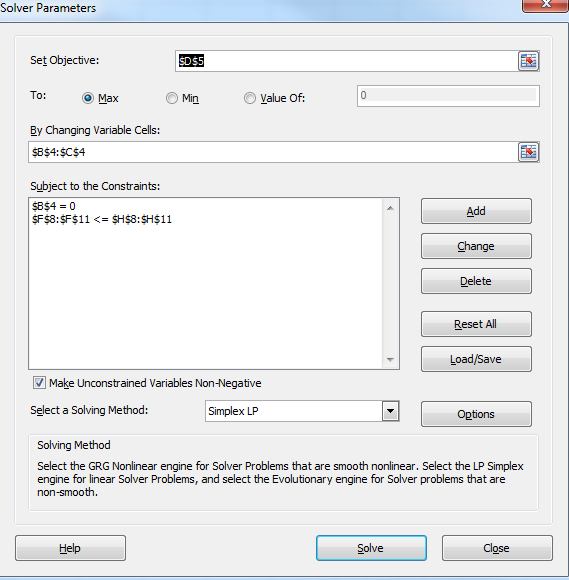The solver produces the following result: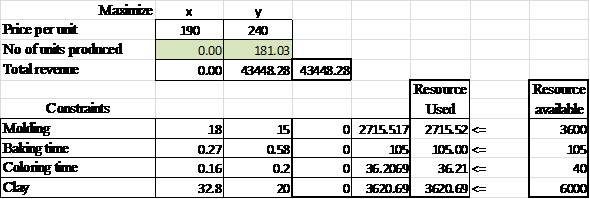Thus 181 batches of smaller tiles must be produced to maximize the profit. G) The sensitivity ranges for the objective function and the constraints are as shown below:h) Formulate the linear programming as shown below: \$190x + \$240y Subject to the following constants: 16x + 12y = 3600 0.27x + 0.58y = 105 0.16x + 0.20y = 40 32.8x + 20y = 6000 Enter the values in the excel sheet as shown below: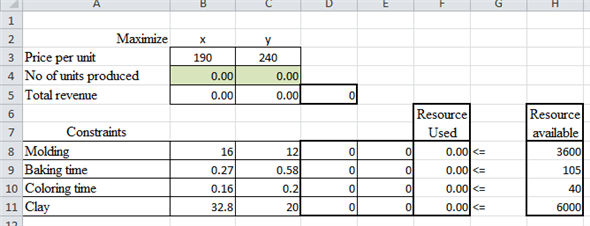Access 'Solver' from the 'data' menu in the excel sheet as shown below:Enter the objectives and the constraints in the solver as shown below:The solver produces the following result: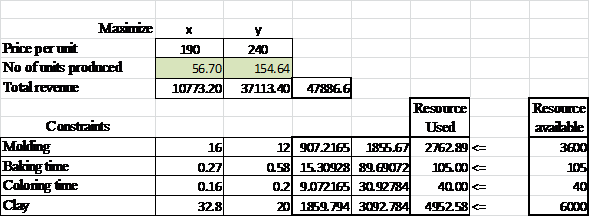Thus, 57 batches of larger tiles and 155 batches of smaller tiles must be produced to maximize the profit. The optimal solution remains the same. I) No, increasing the total pounds of clay wouldn't affect the optimal solution due to its sensitivity range. j) Increasing 20 hours of glazing time would increase the overall profit to 51,376.98.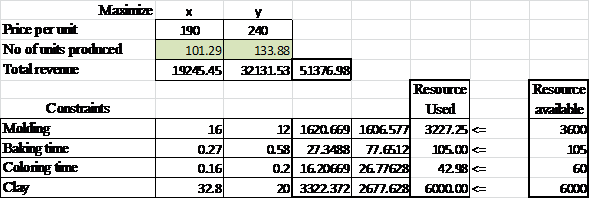The investment is higher than profit earned. Thus it should apt this decision. k) It doesn't affect the optimal solution as the decrease lies within the sensitivity range of 3.7 hours.

Prepare the graphical solution for the problem as shown below: Initially prepare the linear programming model using the mentioned values, as shown below: Objective: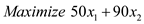Constraints: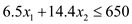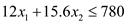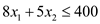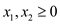Now, replace the inequalities signs with the "equal to" sign in above equations, as shown below: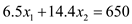…… (1)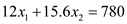…… (2)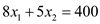…… (3) Now, prepare a graph using the mentioned equations, as elaborated below: Put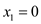in equation (1), it gives the following values:Again, put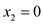in equation (1), it gives the following values:Hence, two points are obtained from the equation namely A (0, 45.14) and B (100, 0). Prepare a line by joining the two points. Using above steps, identify points for each equation and plot the lines accordingly. It would provide the following results: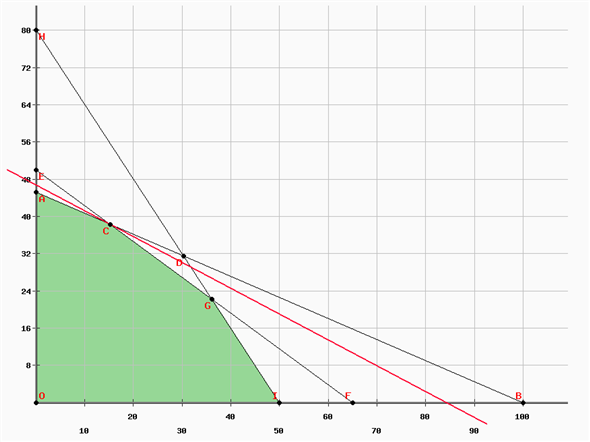Obtain the coordinate for points C, D and G, as shown below: Point C is present at the intersection of equations (1) and (2). Therefore, values of coordinate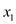and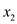would be same for both the lines. Therefore, obtain the value ofby equating the equations for both lines, as shown below: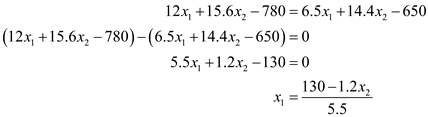Now, put the value ofin equation (1) to obtain the value ofas shown below:Substituting the value ofin equation (1), would give the following result:Hence, the coordinates for point C is (15.30, 38.23). Similarly obtain the other points. Put the values of different point present in the graph in the objective function to determine the optimal solution: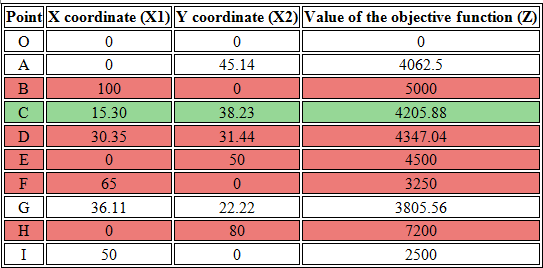Note that the points present in rows highlighted with red ink are present in the infeasible reason. For example, for point B thecoordinate is 100. But putting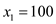in equation (1) would extend its value beyond the feasibility range. Therefore, such points are not included in the feasibility reason. From rest of the values ofand, point C provides the maximum value of the objective function. Therefore, the optimal value ofandwould be 15.30 and 38.23. The value of objective function is 4205.88. Slight differences in the values are due to the rounded off figures. Now, put the values ofandin constraints (1), (2), and (3). It gives the following values: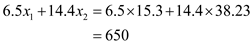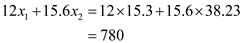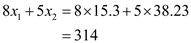Comparing the obtained values with the constraints, it is clear that there is no slack for equation (1) and (2). However, the value of constraint (3) is 314 that is 86 units less than 400. Hence, the third constraints involves a slack of 86.